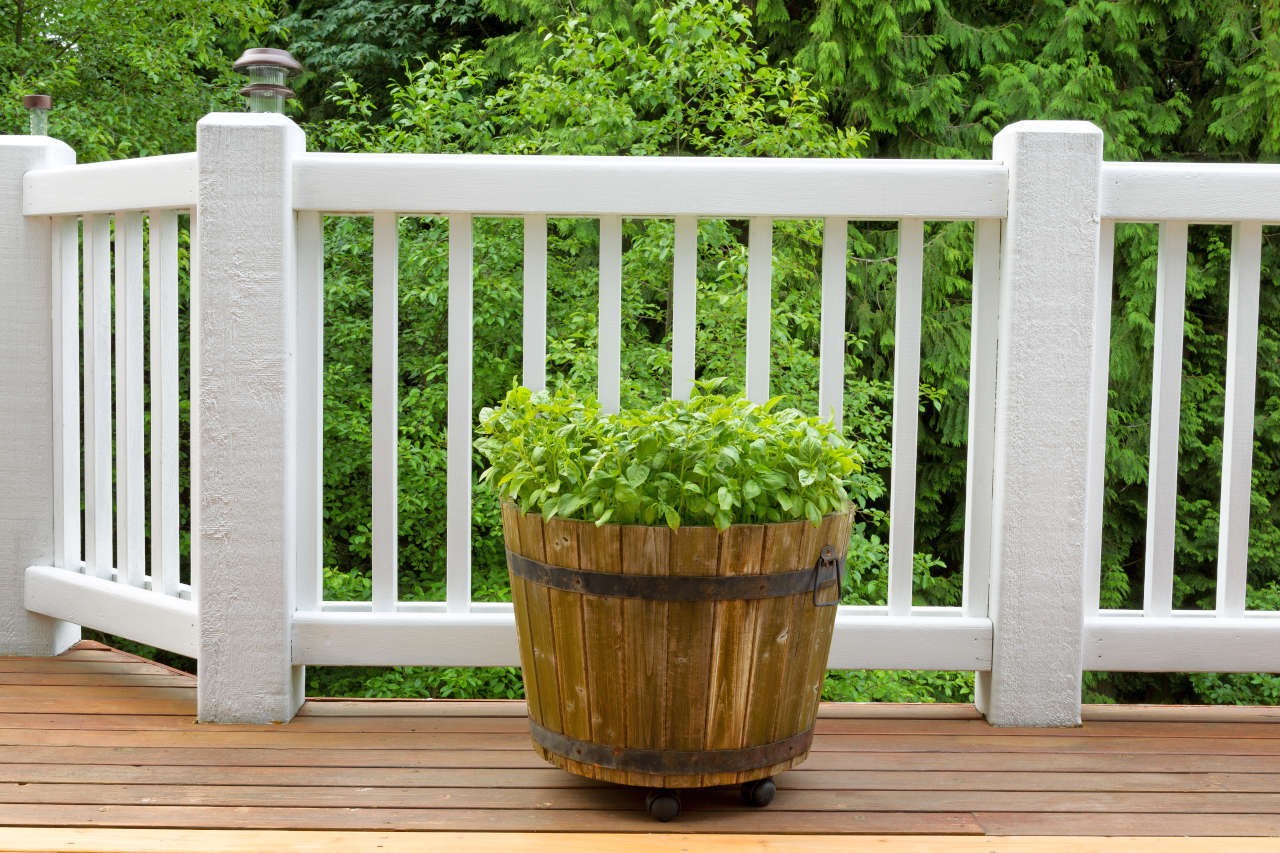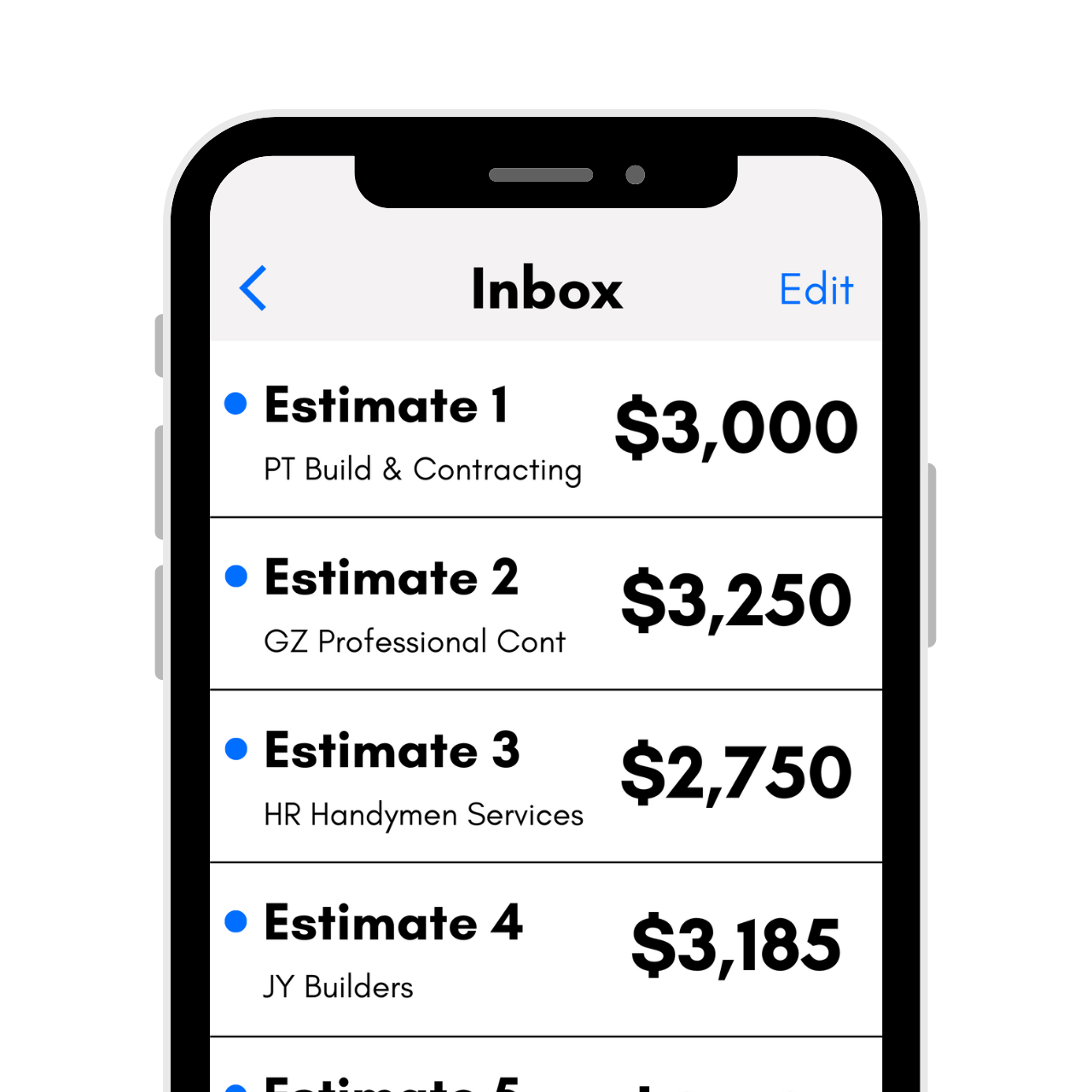# Baluster Calculator

Calculate how many balusters you’ll need for a deck or stair railing by selecting the total railing length, the number and size of posts, and the size of the balusters.

Calculate baluster spacing and layout by entering the baluster size and the length of each section of the railing.

Layout Using:
The maximum safe/legal baluster spacing is 4" in most areas, always follow local building codes for safety.
The maximum safe/legal baluster spacing is 4" in most areas, always follow local building codes for safety.
Railing Sections
Length is the distance between the inside edge of each post.

## Baluster Estimate:

Balusters Needed
Learn how we calculated this below

## How to Calculate How Many Railing Balusters You Need

The railing that goes around your deck or porch is likely made up of evenly spaced balusters or spindles. While the terms are often used interchangeably, these are two different things.Balusters extend straight to the floor or step, while spindles are held in place at the bottom with a horizontal rail. Both, however, are necessary for safety, and are calculated the same way.

You can estimate how many balusters you need for a railing using the calculator above or by following the steps below. If you’re building a deck, check out our deck flooring calculator to estimate the flooring needed for your deck.

To get a quick rough estimate of the number of balusters needed, multiply the total railing length in linear feet, then multiply that by three since the average rail will have roughly three balusters or spindles per running foot, assuming a 4-inch spacing. However, balusters can come in different thicknesses, and can be spaced more closely together if desired.

Get free estimates from deck pros in your area to compare prices### Steps to Estimate Balusters and Spindles

Step One: Measure the Railing Length. The first step to estimating how many balusters you need for a railing is to measure the railing length, making sure to include the stairs as well as all straight areas. If your deck has a railing on either side of the stairs, make sure to account for the second railing.

Step Two: Determine How Many Posts Your Need. You will also need to identify the number of railing posts needed and the thickness of the posts.

To account for the post thickness, simply multiply the number of posts by the thickness, then subtract from the railing length. For example, a 10′ railing with 3 3½”(4×4) posts would have a total length of 120″ – (3 × 3½”) = 120″ – 10.5″ = 109.5″.

Step Three: Account for Spacing Between Balusters/Spindles. The next step in estimating the number of balusters needed is to add the thickness of the baluster or spindle to the maximum space between them to get the combined width. For example, a 1½” (2×2) thick baluster with a 4″ spacing between the balusters would have a combined width of 4″ + 1½” = 5½”.

Note that most lumber sizes are nominal, meaning that a 2×2 baluster will rarely actually measure 2″ in size. Always use exact measurements and not the dimensional lumber size. You can learn more about the true size of dimensional lumber.

Keep in mind as well that spindles and balusters can taper, meaning that they can be a different thickness at the bottom than they are at the top. To maintain a proper 4″ spacing at the thinnest part of the spindles, use the thinnest measurement for your calculations.

Step Four: Calculate the Number of Balusters/Spindles Needed. The final step in finding how many balusters you need is to divide the railing length(minus the posts) from the first step by the combined baluster/spacing width from the second step.

For example, we would take the 109.5″ railing length and divide by 5½”, e.g. 109½” ÷ 5½” = 19.9. Since we can’t add partial balusters, round up the solution from 19.9 to 20.

It is a good idea to order about 10% additional materials to account for waste or measurement errors. This is a general rule of thumb for many construction projects. In the case of the example project above, that would mean ordering one additional baluster.

## How Far Apart Should Balusters Be Spaced?

In most areas, building codes require the maximum spacing between balusters to be 4″ or less for safety. International Residential Code requires a maximum baluster spacing of four inches.

It is important to understand the building code in your area, and safety is an important consideration. We recommend a maximum spacing of 4″ between balusters for this reason.